# WA Arbitrary Path Decomposition VI

LabVIEW 2014 Advanced Signal Processing Toolkit Help

Edition Date: June 2014

Part Number: 372656C-01

»View Product InfoDownload Help (Windows Only)

Owning Palette: Discrete Wavelet VIs

Requires: Advanced Signal Processing Toolkit

Performs subband decomposition by cascading the lowpass analysis filters and the highpass analysis filters and applies a decimation factor of 2 after each filtering step. path specifies the subband and determines how to cascade the lowpass analysis filters and the highpass analysis filters. Wire data to the signal input to determine the polymorphic instance to use or manually select the instance.

Use the pull-down menu to select an instance of this VI.

 Select an instance WA Arbitrary Path Decomposition (Waveform)WA Arbitrary Path Decomposition (Array)

## WA Arbitrary Path Decomposition (Waveform)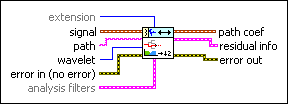extension specifies the method to use to pad data at the borders of the input signal. The extension length is equal to the length of the wavelet filters. When you select the extension method, make the transition between the input signal and the padded data as smooth as possible because a smooth transition generates fewer large detail coefficients and enhances the efficiency of the signal representation.

 0 Zero padding—Uses zeros to pad the input data. Watch for abrupt transitions between the padded zeros and the input data, which causes large artifacts near the transition. 1 Symmetric (default)—Uses replications of the input data to pad the data, except that this VI left-flips the block at the input and right-flips the block at the end. 2 Periodic—Adds a replication of the input data block before and another replication after the input data block to pad the data.signal specifies the input signal.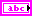path specifies the subband and how to cascade the lowpass analysis filters and the highpass analysis filters. path is a combination of the characters 0 and 1, where 0 represents lowpass filtering, and 1 represents highpass filtering. For example, a value of 101 indicates that this VI passes the signal through a highpass filter, through a lowpass filter, and then through a highpass filter. Use the wavelet input or the analysis filters input to specify the analysis filters.wavelet specifies the wavelet type to use for the discrete wavelet analysis. The default is db02. The options include two types: orthogonal (Haar, Daubechies (dbxx), Coiflets (coifx), Symmlets (symx)) and biorthogonal (Biorthogonal (biorx_x), including FBI (bior4_4 (FBI))), where x indicates the order of the wavelet. The higher the order, the smoother the wavelet. The orthogonal wavelets are not redundant and are suitable for signal or image denoising and compression. The biorthogonal wavelets usually have the linear phase property and are suitable for signal or image feature extraction. If you want to use other types of wavelets, do not wire this input. Instead, use the Wavelet Design Express VI to design the wavelet you want and wire the resulting analysis filters to the analysis filters input.error in describes error conditions that occur before this node runs. This input provides standard error in functionality.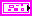analysis filters specifies the coefficients of the lowpass analysis filters and the highpass analysis filters for the wavelet you specify. If you specify a value for analysis filters, this VI ignores the settings in the wavelet input. You can use the Wavelet Design Express VI to design the analysis filters and the corresponding synthesis filters.lowpass specifies the coefficients of the lowpass analysis filter, which this VI uses to compute the approximation coefficients.highpass specifies the coefficients of the highpass analysis filter, which this VI uses to compute the detail coefficients.path coef returns the coefficients of the subband that path defines.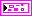residual info returns the information about the complementary subbands at each level. The residual paths describe these complementary subbands. For example, when path is 101, the residual paths include path 0, path 11, and path 100. The WA Arbitrary Path Reconstruction VI uses the complementary subbands information for signal reconstruction.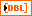coef returns the coefficients on the residual paths. For example, when path is 101, coef includes the coefficients on path 0, path 11, and path 100.length returns the length of the coefficients on the residual paths and the signal length. For example, when path is 101, length includes the signal length and the lengths on path 0, path 11, and path 100.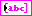residual path returns the residual paths of path.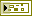error out contains error information. This output provides standard error out functionality.

## WA Arbitrary Path Decomposition (Array)extension specifies the method to use to pad data at the borders of the input signal. The extension length is equal to the length of the wavelet filters. When you select the extension method, make the transition between the input signal and the padded data as smooth as possible because a smooth transition generates fewer large detail coefficients and enhances the efficiency of the signal representation.

 0 Zero padding—Uses zeros to pad the input data. Watch for abrupt transitions between the padded zeros and the input data, which causes large artifacts near the transition. 1 Symmetric (default)—Uses replications of the input data to pad the data, except that this VI left-flips the block at the input and right-flips the block at the end. 2 Periodic—Adds a replication of the input data block before and another replication after the input data block to pad the data.signal specifies the input signal.path specifies the subband and how to cascade the lowpass analysis filters and the highpass analysis filters. path is a combination of the characters 0 and 1, where 0 represents lowpass filtering, and 1 represents highpass filtering. For example, a value of 101 indicates that this VI passes the signal through a highpass filter, through a lowpass filter, and then through a highpass filter. Use the wavelet input or the analysis filters input to specify the analysis filters.wavelet specifies the wavelet type to use for the discrete wavelet analysis. The default is db02. The options include two types: orthogonal (Haar, Daubechies (dbxx), Coiflets (coifx), Symmlets (symx)) and biorthogonal (Biorthogonal (biorx_x), including FBI (bior4_4 (FBI))), where x indicates the order of the wavelet. The higher the order, the smoother the wavelet. The orthogonal wavelets are not redundant and are suitable for signal or image denoising and compression. The biorthogonal wavelets usually have the linear phase property and are suitable for signal or image feature extraction. If you want to use other types of wavelets, do not wire this input. Instead, use the Wavelet Design Express VI to design the wavelet you want and wire the resulting analysis filters to the analysis filters input.error in describes error conditions that occur before this node runs. This input provides standard error in functionality.analysis filters specifies the coefficients of the lowpass analysis filters and the highpass analysis filters for the wavelet you specify. If you specify a value for analysis filters, this VI ignores the settings in the wavelet input. You can use the Wavelet Design Express VI to design the analysis filters and the corresponding synthesis filters.lowpass specifies the coefficients of the lowpass analysis filter, which this VI uses to compute the approximation coefficients.highpass specifies the coefficients of the highpass analysis filter, which this VI uses to compute the detail coefficients.path coef returns the coefficients of the subband that path defines.residual info returns the information about the complementary subbands at each level. The residual paths describe these complementary subbands. For example, when path is 101, the residual paths include path 0, path 11, and path 100. The WA Arbitrary Path Reconstruction VI uses the complementary subbands information for signal reconstruction.coef returns the coefficients on the residual paths. For example, when path is 101, coef includes the coefficients on path 0, path 11, and path 100.length returns the length of the coefficients on the residual paths and the signal length. For example, when path is 101, length includes the signal length and the lengths on path 0, path 11, and path 100.residual path returns the residual paths of path.error out contains error information. This output provides standard error out functionality.

## WA Arbitrary Path Decomposition Details

The following illustration shows an example of an arbitrary path decomposition with the path input set to 101 and the extension input set to Periodic. The length of the input signal is 16 points.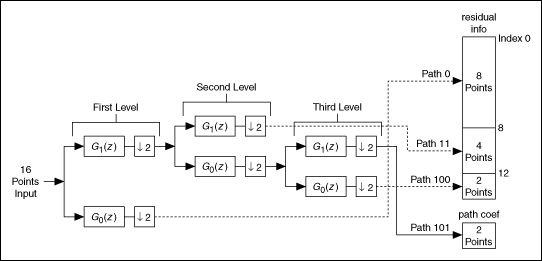G1(z) denotes that the signal passes through a highpass filter. G0(z) denotes that the signal passes through a lowpass filter. G1(z) and G0(z) form the analysis filter bank.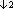denotes a decimation on the signal with a factor of 2. Path 101 indicates that this VI passes the signal through a highpass filter G1(z), through a lowpass filter G0(z), and then through a highpass filter G1(z).

Using information in the previous illustration, you can see that the residual info output contains the information about the complementary subbands at each level, and you can see that the path coef output of this VI contains the coefficients of the subband that path defines.

A discrete wavelet decomposition decomposes the lowpass filtering output, or the approximation coefficients, at each level. However, an arbitrary path decomposition does not restrict the decomposition to the lowpass filtering output. You can use the lowpass filtering output or the highpass filtering output for the further decomposition.

The residual info output stores the information of path 0, path 11, and path 100 in the above example for reconstruction. You can use the source coefficients input of the WA Arbitrary Path Reconstruction VI to select path coefficients and/or residual coefficients to reconstruct the signal.

## Example

Refer to the Engine Knocking Detection VI in the labview\examples\Wavelet Analysis\WAApplications directory for an example of using the WA Arbitrary Path Decomposition VI.

Not Helpful